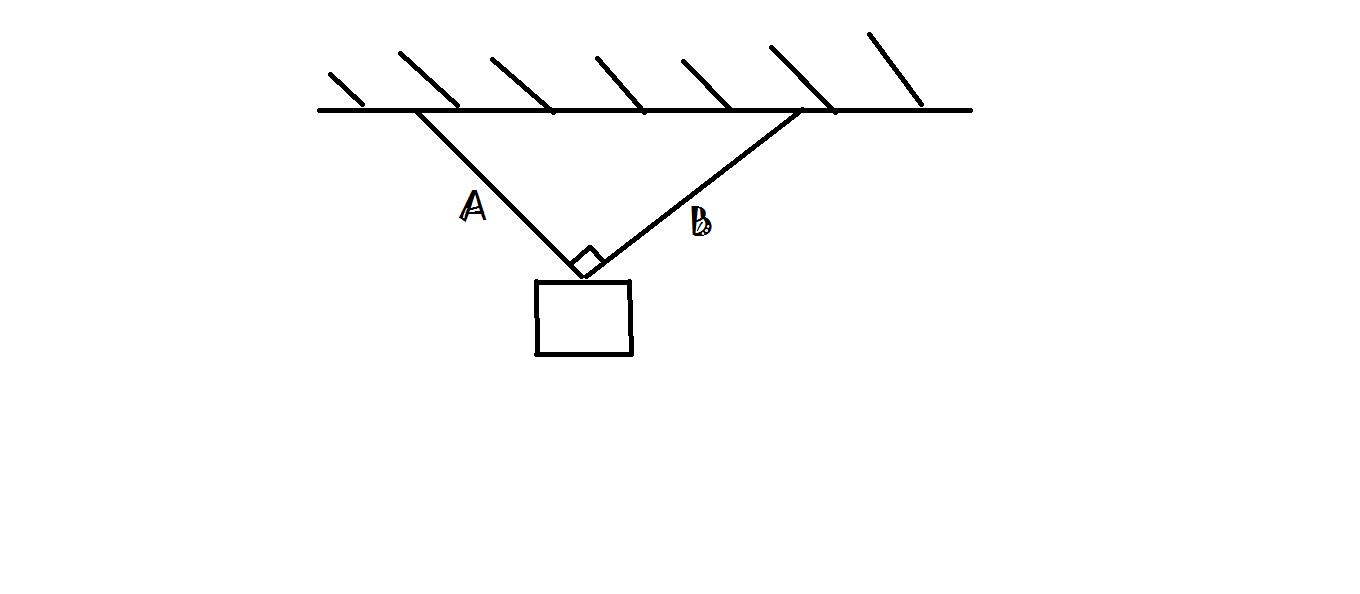# A classical mechanics problem by Pankaj JoshiAn object is hanged from ceiling with help of two light strings A & B. Object stays in equilibrium with tension in A & B equal to 40N and 30N respectively. Now the object is pulled by a constant force F=120N perpendicular to the initial plane of object and two strings. If object stays in equilibrium position. Find the tension in string B.

• Take $g = 10 m/s^2$
×